INSTEP STUDIO 3D

# Creating additional Prismatic Elements: The Inflate Tool

Time to read: ~4 min

The Inflate tool allows Solid Elements to grow using Prismatic Elements or for Shell Elements to be converted to (multiple) Solid Elements.

Inflation of Shell elements or Solid Elements are aimed at supporting workflows where either an existing mesh is to be augmented (such as where a solid mesh is generated for a structural FE solver but is now to be used with a solver that requires better resolution near the surface) or where a Shell/Sheet type mesh is to be used in an application that only supports Solid Elements.

The workflow will depend on whether the source data consist of Solid Elements that are to be expanded or whether the data is made up of Shell Elements that are to be inflated/thickened into Solid Elements.

## From Shell Body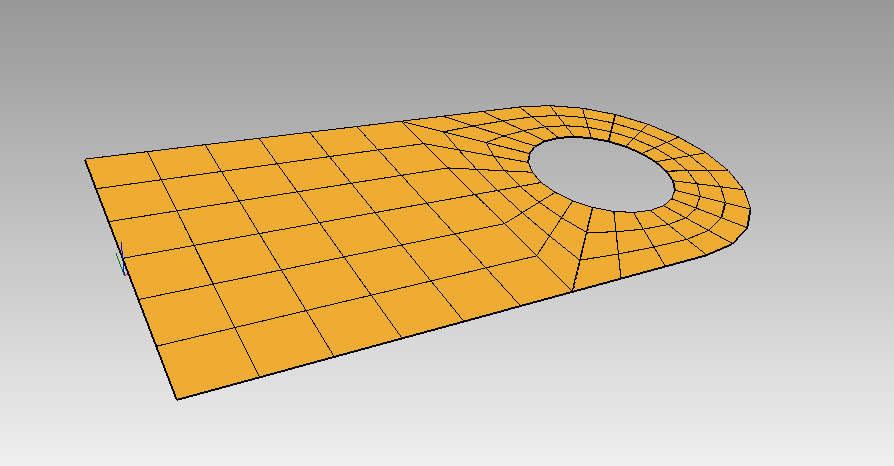If the starting data is a Shell Body, then the option (in the sidebar under the 'Inflate' tab) to use is the 'Thicken Surfaces'. This method allows Prismatic elements to be created from the Shell elements along the normals of their faces. It is important that the Elements' face orientation is correct, otherwise the growth of the elements can be unpredictable.
If the number of Layers is set to an Odd number and the option to 'Apply to Both Sides' is set, then the first layer generated extends half way on either side of the originals: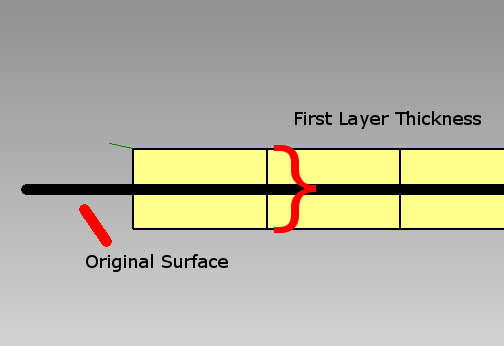Single Inflation Layer from Shell elements applied to both sides

For the case where more than one layer is to be generated (and the number of layers is still an odd number), the resultant layers are distributed equally on both sides: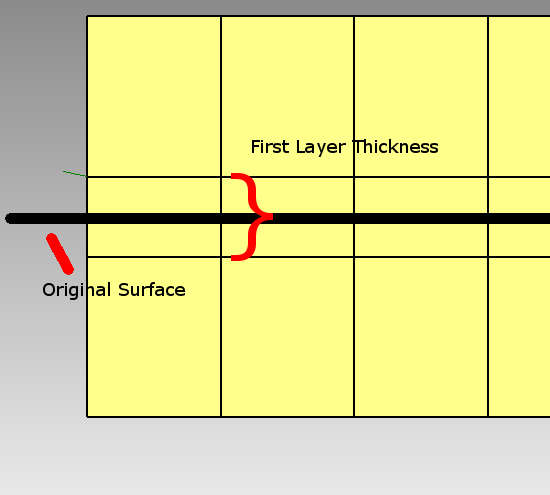Three Inflation Layers applied to both sides of Shell Elements

In the case where there are an even number of layers to be generated, the offset (Applied to Both Sides) is equal but the original Shell elements now lie between the first and second layer: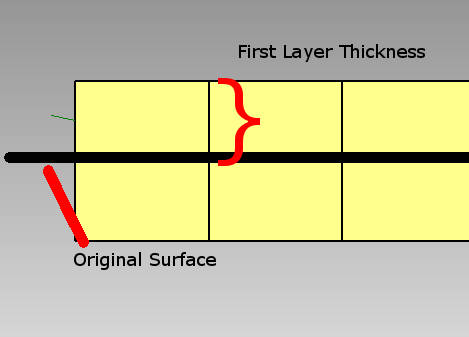Two Inflation Layers equally applied to either side of Shell Elements

## From Solid Body

For the case where the input data contains Solid Elements, the option to 'Inflate Solid Surfaces' is appropriate. This approach generates prismatic elements on top of the exterior surfaces of a solid body, essentially growing into unused/undefined space: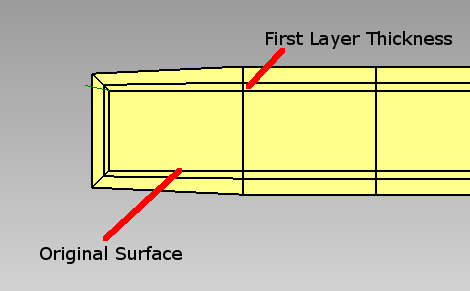Inflation using Inflate Solid Surfaces Option

In this view, the interior elements are made visible by generating a Mid-Plane through the body after inflation. What is to be pointed out is that the inflation will always proceed along the normal vector computed as the average of the Nodes' attached surfaces. In this case, the normal directions in the corners are the average of the top and side surfaces and thus the extension is at an angle.

## Layers

The number of Layers is the number of iterations during which to iteratively expand the mesh. A value of 1 means that there will be one layer of elements added. After each layer has been added, the local normal vectors are recomputed which helps (but does not necessarily prevent) internal pockets from becoming distorted or overlapping.

## First Layer Thickness

The amount of offset that is to be applied to the newly formed Nodes is a factor of the normal vector (with a length equal to 1). Unlike the Thicken tool for the Polygon-Body data, the amount of offset is to be uniform and not dependent on local effects. If only one layer is to be generated, then the thickness of this layer is equal to this value. If multiple layers are being generated, the each layer thickness is a function of the first layer, the layer growth rate and the number of layers (see below).

## Growth Rate

The thickness of each layer is a function of the first layer thickness, growth rate and number of layers. The thickness of layer 'n' is 1st_layer_thickness*(growth_rate^(n-1)) so for the first layer this is simply 1st_layer_thickness. For the second layer (n=1), this is 1st_layer_thickness*growth_rate for the 3rd layer (n=2), this is 1st_layer_thickness*growth_rate^2. From this it can be seen that a value for Growth Rate between 0 and 1 makes each following layer thinner than the previous and values larger than 1 make them larger. A value of 1 means that each layer is the same thickness.